### Ticker

6/recent/ticker-posts

# How "Q-factor" reflects the quality of a digital optical communications signal.

Q-factor:  How reflects the quality of a digital optical communications signal. Telecom friends always discuss or talk about the Q-factor but mostly not familiar with the concept of Q-factor. Today I will try to write about Q-factor in very simple way.

What is the Q-factor :The Q-factor is a parameter that directly reflects the quality of a digital optical communications signal.The higher the Q-factor,the better the quality of the optical signal.Q-factor measurement is related to the analog signal and in this respect differs from traditional BER tests. As the Q-factor is related to the analog signal it gives a measure of the propagation impairments caused by optical noise,non-linear effects,polarization effects and chromatic dispersion.Q-factor details from www.technopediasite.com

How "Q-factor" reflects the quality of a digital optical communications signal

Here we are discussing about the Q-factor. How Q-factor is important for the digital optical communications signal & what is role in digital optical communications signal.

Propagation impairments caused by optical noise,non-linear effects,polarization effects and chromatic dispersion.Impairments for optical systems can be categorized into linear and non-linear effects. But here i don't like to explain about the linear & non-linear effects.
The Q-factor is a measure for the quality of the analog signal, which is usually defined by its signal-to-noise ratio (this is also the mathematical definition of the Q-factor). The Q-factor is defined by the difference of the mean values of the two signal levels divided by the sum of the noise rms values (standard deviations) at the two signal levels.This can be expressed by the following equation.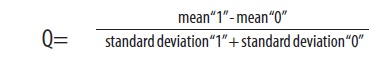Q-factor equation

The analog representation of a digital signal on the time scale toggles between the states “0”and “1”depending on the data pattern to be sent.Data pattern

As the illustrations show the signal is more likely to be at the “0”or “1”level rather than

in a transient state. Real signals are also influenced by noise which causes the most likely amplitude levels to spread out.

Optical eye with optimum detection point
The detection of the binary level during the pulse width is not only dependent on
the detection threshold but also on the detection time.The detection time is often
expressed as sampling phase.This comes from the definition of the pulse width in

radians. The pulse width itself may be defined to cover the range from 0 to 2. see in below figure.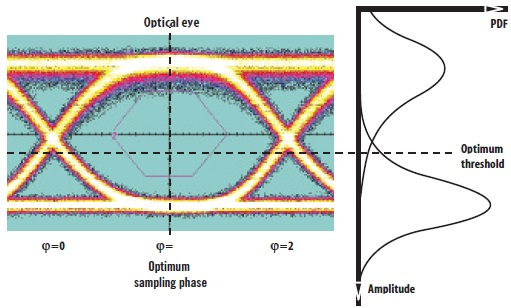Optical eyes

The center of the eye opening provides optimal conditions for the detection of the binary signal and is defined as the sampling phase with the highest vertical eye opening.
Now understand how Q-factor relates to the BER.The difference in the mean values produces the vertical eye opening.The higher the difference,the better the BER will be as the two bell curves drift away from each other and have less overlap.This difference is divided by the sum of the noise distributions which are represented by the width of the bell curves.Increases in noise result in more overlap in the two bell curves resulting in a higher BER.

Methods to determine the Q-factor

Two fundamental methods for determining the Q-factor:
1. The histogram method
2. The Pseudo-BER method

Fundamental method                     Sampling                       Description

Histogram method                           Asynchronous                   Voltage histogram                                                                            Synchronous                     Digital sampling scope

Pseudo-BER method                      Synchronous                     Single threshold method                                                                  Synchronous                     Dual threshold method
Asynchronous sampling (voltage histogram)-All the amplitude values of the eye diagram (including the amplitude values of the transient regions) are sampled asynchronously resulting in a histogram representing the PDF of the complete signal including the transient regions.For more details please see the below figure.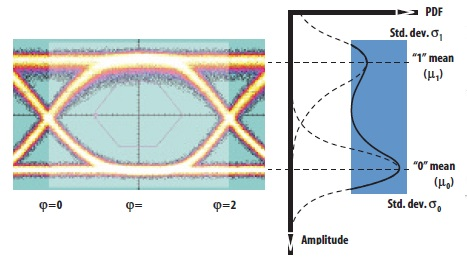Voltage Histogram

Synchronous sampling (digital sampling scope)-The main restriction of asynchronous sampling is that the transient regions affect the result.To overcome this restriction and gain higher accuracy,synchronous sampling must be performed. Synchronous sampling needs a clock recovery and is therefore more complex.The sampling phase is locked to the optimum phase and can therefore give a more accurate result of the BER estimation.Synchronous sampling concentrates more on the detection phase rather than the whole signal.

One disadvantage of this method is that the digital sampling scopes used often do not have the sampling rate needed for Q-factor measurements. A typical sampling rate would be 100,000 samples per second.Assuming a 10 Gbps signal (10,000,000,000 bits per second) is received,only one bit out of 100,000 would be sampled.

Synchronous sampling method (single decision threshold method) –Rather than taking the histogram to determine the shape of the PDF (and thus the estimated BER), BER measurements at different decision threshold levels can be taken to extrapolate
the estimated BER.

By taking decision threshold levels which are correlated to BERs of 10-4 to 10-8,the measurement can be completed much quicker below table. Assuming the distribution of the PDFs are Gaussian,the BER of the optimum threshold level can be evaluated giving an estimated BER as opposed to an actual measured value.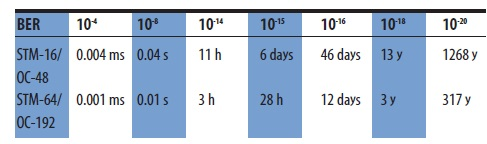table

By using the Pseudo-BER method,the number of samples is the same as those given by the bit rate.For example,a 10 Gbps signal results in 10,000,000,000 samples per second,which when compared to a sampling scope gives a much higher rate.

Synchronous sampling method (dual decision threshold method) –The single decision threshold method is based on the traditional BER testing with a known bit pattern (PRBS).This of course has the drawback that the single decision threshold method can only be performed in out-of-service mode.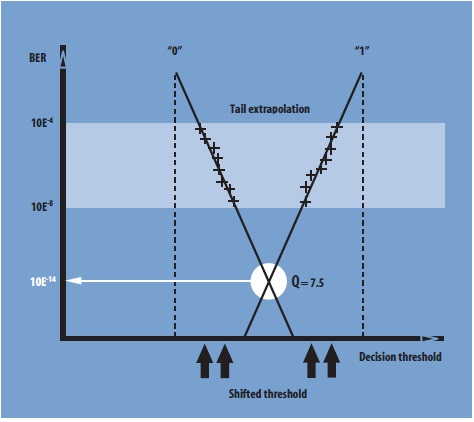Q-factor extrapolation

Each threshold level generates a data point in the range of 10-4 to 10-8 in order to receive short measurement time.An extrapolation using the measured BERs (light area in figure 24) allows for an estimation of the BER of the optimum threshold level (Q-factor point in above figure).The estimated BER can be expressed as Q-factor.

Q-factor applications

Manufacturing-System components must be checked after manufacturing.
Installation- functionality of equipment set up at network operator
sites must be verified-functionality of equipment set up at network operator sites must be verified
Optimization-systems currently in operation must be optimized for best system performance.
Maintenance and roubleshooting-Covering tasks where the Q-factor meter can be used as a measuring tool.
Monitoring-Q-factor can show the smallest of signal degradation.

Conclusions

The Q-factor meter estimates the BER for optimum threshold level and sampling
phase in less than 1 minute,making the Q-factor measurement faster than traditional
BER testing.The Q-factor measurement is independent of bit rate and data format and as such is a “universal tool”in systems carrying different bit rates and protocols.As DWDM systems are transparent for different data formats the Q-factor meter is an ideal solution.In addition to this,the Q-factor does not require a known bit pattern to be sent thus allowing in-service monitoring when appropriate tapped signals are provided.